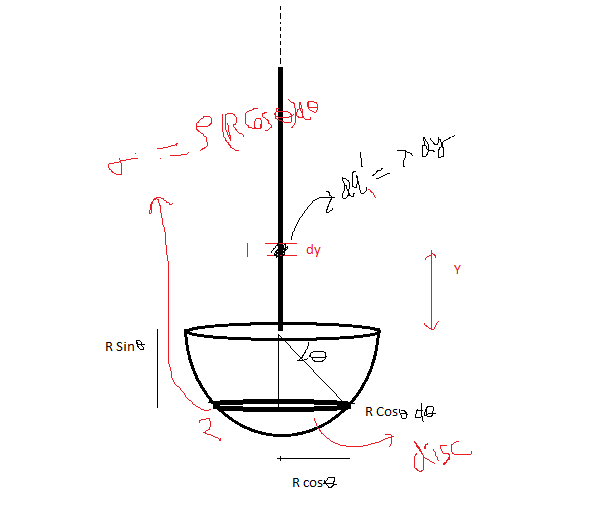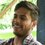# Jatin Yadav's "Force of interaction"Recently I solved an question of @Jatin Yadav Force of interection Click here

In this question I calculate Force of interaction by First Calculating Electric field due to long wire and then calculate force between them ( Which is Well explained in Jatin's Solution ). I had also solved that in this way First.

Doubt

In That Question If we consider Electric field due disc element in hemisphere and then Find The Force of interaction then How We can calculate This ?

My WORK

Consider an disc element on Hemisphere and small line element on wire as shown in figure . Sorry For poor Diagram But please Feel it Now I use standard result of electric field due to an uniformly charged disc that is

$\displaystyle{{ E }_{ at\quad x }\quad =\quad \cfrac { \sigma }{ 2\epsilon } (1\quad -\quad \cfrac { x }{ \sqrt { { x }^{ 2 }+{ R }^{ 2 } } } )}$.

$\displaystyle{{ F }_{ 1,due\quad to\quad 2 }\quad =\quad \int { { dE }_{ 2 }{ dq }_{ 1 } } \quad \quad (\quad along\quad y-axis\quad )\\ \quad \quad \quad \\ \because \quad { dq }_{ 1 }\quad =\quad \lambda dy\\ \\ \because \quad \sigma =\quad \rho R\cos { \theta d\theta } \quad \quad \\ \\ \therefore \quad { dE }_{ 2 }\quad =\quad \cfrac { \quad \rho R\cos { \theta d\theta } }{ 2\epsilon } (1\quad -\quad \cfrac { y+R\sin { \theta } }{ \sqrt { { (y+R\sin { \theta } ) }^{ 2 }+{ (R\cos { \theta } ) }^{ 2 } } } )\\ \\ \boxed { { F }_{ 1,due\quad to\quad 2 }\quad =\quad \cfrac { \rho \lambda R }{ 2\epsilon } \int _{ y=0 }^{ y=\infty }{ \int _{ \theta =0 }^{ \theta ={ \pi }/{ 2 } }{ \cos { \theta (1\quad -\quad \cfrac { y+R\sin { \theta } }{ \sqrt { { (y+R\sin { \theta } ) }^{ 2 }+{ (R\cos { \theta } ) }^{ 2 } } } )d\theta } dy } } } }$.

But From Here I get stuck

How can I find this integral ..??

Also When I use wolfram alpha then it says this this Integral not converges.!!

So please Tell Me where I'am wrong. ??

Thanks!!Note by Deepanshu Gupta
6 years, 7 months ago

This discussion board is a place to discuss our Daily Challenges and the math and science related to those challenges. Explanations are more than just a solution — they should explain the steps and thinking strategies that you used to obtain the solution. Comments should further the discussion of math and science.

When posting on Brilliant:

• Use the emojis to react to an explanation, whether you're congratulating a job well done , or just really confused .
• Ask specific questions about the challenge or the steps in somebody's explanation. Well-posed questions can add a lot to the discussion, but posting "I don't understand!" doesn't help anyone.
• Try to contribute something new to the discussion, whether it is an extension, generalization or other idea related to the challenge.
• Stay on topic — we're all here to learn more about math and science, not to hear about your favorite get-rich-quick scheme or current world events.

MarkdownAppears as
*italics* or _italics_ italics
**bold** or __bold__ bold
- bulleted- list
• bulleted
• list
1. numbered2. list
1. numbered
2. list
Note: you must add a full line of space before and after lists for them to show up correctly
paragraph 1paragraph 2

paragraph 1

paragraph 2

[example link](https://brilliant.org)example link
> This is a quote
This is a quote
    # I indented these lines
# 4 spaces, and now they show
# up as a code block.

print "hello world"
# I indented these lines
# 4 spaces, and now they show
# up as a code block.

print "hello world"
MathAppears as
Remember to wrap math in $$ ... $$ or $ ... $ to ensure proper formatting.
2 \times 3 $2 \times 3$
2^{34} $2^{34}$
a_{i-1} $a_{i-1}$
\frac{2}{3} $\frac{2}{3}$
\sqrt{2} $\sqrt{2}$
\sum_{i=1}^3 $\sum_{i=1}^3$
\sin \theta $\sin \theta$
\boxed{123} $\boxed{123}$

## Comments

Sort by:

Top Newest

This integral would give the same answer.

Note that $\displaystyle \int \bigg(1 - \dfrac{y+R \sin \theta}{(y+R \sin \theta)^2 + R^2 \cos^2 \theta}\bigg) {\mathrm dy} = y - \sqrt{(y+R \sin \theta)^2+R^2 \cos^2 \theta}$

which approaches to $R \sin \theta$ at $y = \infty$

$\Rightarrow \displaystyle \int_{0}^{\infty} \bigg(1 - \dfrac{y+R \sin \theta}{\sqrt{(y+R \sin \theta)^2 + R^2 \cos^2 \theta}} {\mathrm dy}\bigg) = R(1- \sin \theta)$

Hence, the integral can be expressed as:

$\displaystyle \dfrac{\rho \lambda R}{2 \epsilon_{0} } \int_{0}^{\pi/2} R(1- \sin \theta) \cos \theta d \theta = \boxed{\dfrac{\rho \lambda R^2}{4 \epsilon_{0}}}$

- 6 years, 7 months ago

Log in to reply

Wow !! Thanks a ton !! for Replying @jatin yadav

I haven't study about the double integration Yet But Now I understand Your Solution . So From your solution I draw The Conclusion That Double integral is nothing but it is calculate by integrating first integral keeping parameters of 2nd Integral Constant and then integrate 2nd Integral ,Is I'am Right ??

Also I would feel good if You will give me some facts about Double Integral (If Possible)

And Isn't There is mistake in typing of latex in starting line of your comment ?

- 6 years, 7 months ago

Log in to reply

Yes you are right , double integral is done in the same way as you said @Deepanshu Gupta

- 6 years, 6 months ago

Log in to reply

Thanks for replying! Lalit

- 6 years, 6 months ago

Log in to reply

×

Problem Loading...

Note Loading...

Set Loading...